Cyclic Convolution Matrix Next  |  Prev  |  Up  |  Top  |  Index  |  JOS Index  |  JOS Pubs  |  JOS Home  |  Search

### Cyclic ConvolutionMatrix

An infinite Toeplitz matrix implements, in principle, acyclic convolution (which is what we normally mean when we just say convolution''). In practice, the convolution of a signal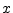and an impulse response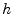, in which bothandare more than a hundred or so samples long, is typically implemented fastest using FFT convolution (i.e., performing fast convolution using the Fast Fourier Transform (FFT) 6.9). However, the FFT computes cyclic convolution unless sufficient zero padding is used . The matrix representation of cyclic (or circular'') convolution is a circulant matrix, e.g.,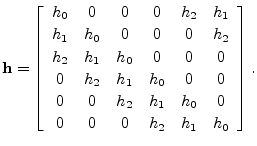As in this example, each row of a circulant matrix is obtained from the previous row by a circular right-shift. Circulant matrices are thus always Toeplitz (but not vice versa). Circulant matrices have many interesting properties.6.10 For example, the eigenvectors of an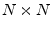circulant matrix are the DFT sinusoids for a length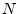DFT . Similarly, the eigenvalues may be found by simply taking the DFT of the first row.

The DFT eigenstructure of circulant matrices is directly related to the DFT convolution theorem . The above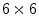circulant matrix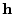, when multiplied times a length 6 vector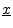, implements cyclic convolution ofwith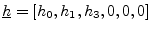. Using the DFT to perform the circular convolution can be expressed asIDFT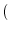DFT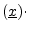DFT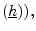where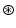' denotes circular convolution. Let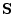denote the matrix of sampled DFT sinusoids for a lengthDFT: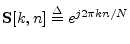. Then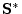is the DFT matrix, where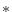' denotes Hermitian transposition (transposition and complex-conjugation). The DFT of the length-vectorcan be written as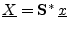, and the corresponding inverse DFT is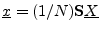. The DFT-eigenstructure of circulant matrices provides that a realcirculant matrixhaving top row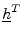satisfies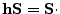diag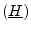, where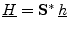is the lengthDFT of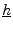, and diagdenotes a diagonal matrix with the elements of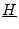along the diagonal. Therefore, diag. By the DFT convolution theorem,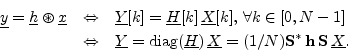Premultiplying by the IDFT matrix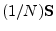yields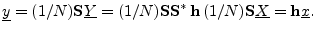Thus, the DFT convolution theorem holds if and only if the circulant convolution matrixhas eigenvaluesand eigenvectors given by the columns of(the DFT sinusoids).

Next  |  Prev  |  Up  |  Top  |  Index  |  JOS Index  |  JOS Pubs  |  JOS Home  |  Search

[How to cite this work] [Order a printed hardcopy]